The Bohr Model

The first successful model of hydrogen was developed by Bohr in 1913, and incorporated the new ideas of quantum theory. The major success of this model was an explanation of the simple formula (28.1) for the emission spectra. The arguments begin with considering the electron orbiting around the proton, as in the planetary model sketched in Fig. 28.1.Such an electron will have a kinetic energy,

 KE =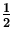mv 2, (3)

and an electrical potential energy

 PE = k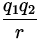= k= - k, (4)

so that the total energy is

 E =mv 2 - k. (5)

Now, from Newton's 2 nd law, we know that the Coulomb force supplies the centripetal acceleration:

 k= m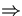mv 2 = k, (6)

so that the total energy of Eq.(28.5) becomes

 E = - k. (7)

Up until this point the analysis has been classical, and as such the model suffers from the instability problem mentioned previously. What Bohr did was to now consider the electron not as a particle but as a wave, with wavelength given by de Broglie's relation: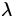=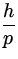=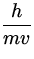. (8)

Furthermore, if one tries to wrap'' such a wave around a circular orbit about the proton, as in Fig. 28.2,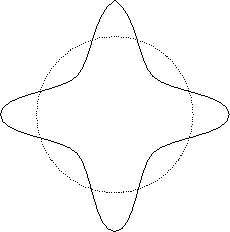then only certain wavelengths would be allowed for a stable orbit:

 2r = n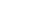n = 1,2,3,... (9)

Combining this with Eq.(28.8), we see that this quantization condition becomes

 mvr = n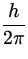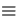n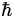, (10)

where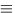h/2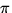. This then says that the angular momentum of the electron is quantized in units of.

If one combines Eqs.(28.6,28.10), one sees

 v 2 ==rn =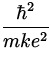n 2a n 2, (11)

where

 a0 == 0.053 nm (12)

is a constant called the first Bohr radius. Thus, in this model only certain radii rn satisfying Eq.(28.11) are allowed for stable orbits. Corresponding to these orbits are discrete energy levels; one finds

 En = --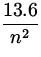eV . (13)

Thus, the electrons in this model are confined to certain orbits with definite energies. Let us consider what happens when an electron in a high energy orbit n = ni falls to a lower energy state with n = nf , where ni > nf . Such a process in itself would not conserve energy; let us postulate that the excess energy is carried away by a photon of energy hf = hc/. Energy conservation then tells us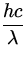+ Eni = Enf=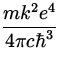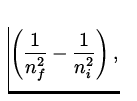(14)

which is of the same form as Eq.(28.1) for the hydrogen spectra, and also gives an expression for Rydberg's constant (28.2) in terms of known quantities:

 RH == 1.097 x 107 m - 1. (15)

This provides excellent agreement with experiment, and was a major success of the early quantum theory.Next: Other Atoms Up: Atomic Physics Previous: Atomic Spectra